# Mathematics 1010 online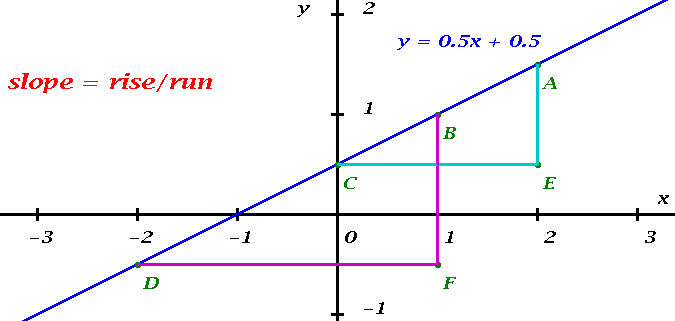## Equations and Graphs of Straight Lines

### Slope

All you need to understand about straight lines is contained in the picture above. Think of moving along the blue line. You climb one unit for every two units that you move to the right. The slope of the line is the ratio of the vertical and the horizontal distances, called rise and run, respectively.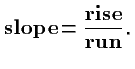A straight line is characterized by the fact that the slope is independent of where you compute the rise and the run. The two triangles AEC and BFD in the Figure are similar. This means that corresponding angles are equal, and hence the ratios of the lengths of corresponding sides are equal. It does not matter which two points on the line we pick (as long as they are distinct), we will get a similar triangle, and the same slope, for all choices. In the picture, the various points have the following coordinates: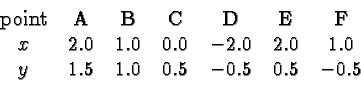Using the cyan triangle AEC we obtain for the slope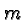of the line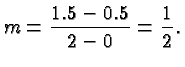Using the magenta triangle BFD we obtain the same value:Everything about straight lines flows from the concept of slope. There are some details and some language that you need to be familiar with. But essentially all problems concerning straight lines, certainly in this class, require that you work with the slope of the straight line.

### The Slope-Intercept Form of a line.

The line in the above Figure is described by the equation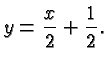Note that this is consistent with what we have observed before. If we increase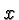by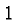thenincreases byand so the slope of that line is. When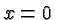thenand so the-intercept is. To obtain the-intercept we set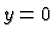and solve for: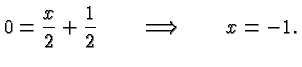These considerations generalize. An equation of the formdefines a line with slopeandintercept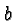. It is called the slope-intercept form of a line.

### The Two-Point-Form of a Straight Line.

Suppose we are given two points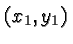and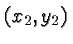on a line. Let's denote a general point on the line by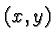. Then, since the slope is independent of the choice of points we obtain the equation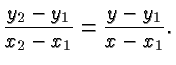This is called the two-point-form of a straight line. For example, for the line in the Figure, picking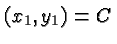and, as indicated in the cyan triangle in the above Figure, we obtain the equation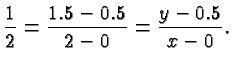Multiplying withand adding 0.5 on both sides gives the slope-intercept form.

### The Point-Slope-Form of a Straight Line.

If we are given the slopeof a line, and a pointon it, we denote again bya general point and obtainThis is called the point-slope form of the line. For example, using againand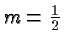we obtain for the line in our Figure: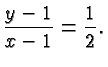Multiplying withand adding 1 on both sides again gives the familiar slope intercept form.

### The general equation of a straight line.

An equation of the form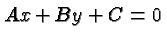where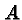,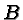and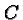are constants defines a straight line. For example, our line on this page can be written aswhere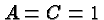and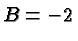. Once again, you can solve forand obtain the slope-intercept form. Such a general equation has the advantage of capturing the possibility of a vertical line (where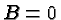).

### Perpendicular Lines

Two lines with slopesand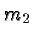are perpendicular if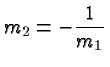or, equivalently,

This makes sense: if one line goes up the other goes down (since their slopes have opposite signs), and if one line is shallow the other is steep.

The following Figure explains why the slopes are related in this way: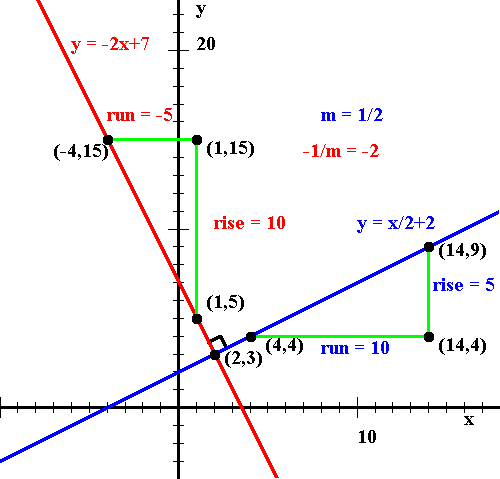It shows two perpendicular lines with slopes 1/2 (shown in blue) and -2 (shown in red), intersecting in the point (2,3). The two green triangles are congruent, and the one associated with the red line can be obtained by rotating by 90 degrees the other triangle associated with the blue line. If you think of subtracting the coordinates of the point close to the intersection from those of the point far away, that rotation turns the original run into the new rise, and the original rise into the negative of the new run, as illustrated in the Figure. You can also think of subtracting the coordinates of the leftmost point from those of the rightmost, in which case the rise is multiplied with negative 1 and the run maintains its sign, without affecting the sign of the slope.